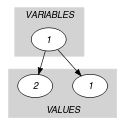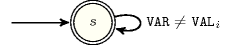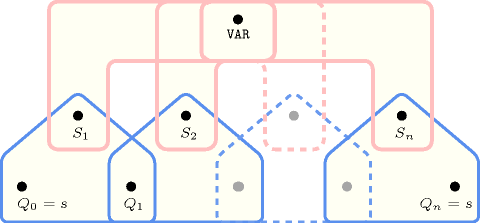## 5.283. not_in

Origin

Derived from $\mathrm{𝚒𝚗}$.

Constraint

$\mathrm{𝚗𝚘𝚝}_\mathrm{𝚒𝚗}\left(\mathrm{𝚅𝙰𝚁},\mathrm{𝚅𝙰𝙻𝚄𝙴𝚂}\right)$

Arguments
 $\mathrm{𝚅𝙰𝚁}$ $\mathrm{𝚍𝚟𝚊𝚛}$ $\mathrm{𝚅𝙰𝙻𝚄𝙴𝚂}$ $\mathrm{𝚌𝚘𝚕𝚕𝚎𝚌𝚝𝚒𝚘𝚗}\left(\mathrm{𝚟𝚊𝚕}-\mathrm{𝚒𝚗𝚝}\right)$
Restrictions
 $\mathrm{𝚛𝚎𝚚𝚞𝚒𝚛𝚎𝚍}$$\left(\mathrm{𝚅𝙰𝙻𝚄𝙴𝚂},\mathrm{𝚟𝚊𝚕}\right)$ $\mathrm{𝚍𝚒𝚜𝚝𝚒𝚗𝚌𝚝}$$\left(\mathrm{𝚅𝙰𝙻𝚄𝙴𝚂},\mathrm{𝚟𝚊𝚕}\right)$
Purpose

Enforce $\mathrm{𝚅𝙰𝚁}$ to be assigned a value different from the values of the $\mathrm{𝚅𝙰𝙻𝚄𝙴𝚂}$ collection.

Example
$\left(2,〈1,3〉\right)$

The constraint $\mathrm{𝚗𝚘𝚝}_\mathrm{𝚒𝚗}$ holds since the value of its first argument $\mathrm{𝚅𝙰𝚁}=2$ does not occur within the collection $〈1,3〉$.

Typical
$|\mathrm{𝚅𝙰𝙻𝚄𝙴𝚂}|>1$
Symmetries
• Items of $\mathrm{𝚅𝙰𝙻𝚄𝙴𝚂}$ are permutable.

• One and the same constant can be added to $\mathrm{𝚅𝙰𝚁}$ as well as to the $\mathrm{𝚟𝚊𝚕}$ attribute of all items of $\mathrm{𝚅𝙰𝙻𝚄𝙴𝚂}$.

Arg. properties

Contractible wrt. $\mathrm{𝚅𝙰𝙻𝚄𝙴𝚂}$.

Remark

Entailment occurs immediately after posting this constraint and removing all values in $\mathrm{𝚅𝙰𝙻𝚄𝙴𝚂}$ from $\mathrm{𝚅𝙰𝚁}$.

Systems

notMember in Choco, rel in Gecode.

Used in
Keywords
Derived Collection
$\mathrm{𝚌𝚘𝚕}\left(\mathrm{𝚅𝙰𝚁𝙸𝙰𝙱𝙻𝙴𝚂}-\mathrm{𝚌𝚘𝚕𝚕𝚎𝚌𝚝𝚒𝚘𝚗}\left(\mathrm{𝚟𝚊𝚛}-\mathrm{𝚍𝚟𝚊𝚛}\right),\left[\mathrm{𝚒𝚝𝚎𝚖}\left(\mathrm{𝚟𝚊𝚛}-\mathrm{𝚅𝙰𝚁}\right)\right]\right)$
Arc input(s)

$\mathrm{𝚅𝙰𝚁𝙸𝙰𝙱𝙻𝙴𝚂}$ $\mathrm{𝚅𝙰𝙻𝚄𝙴𝚂}$

Arc generator
$\mathrm{𝑃𝑅𝑂𝐷𝑈𝐶𝑇}$$↦\mathrm{𝚌𝚘𝚕𝚕𝚎𝚌𝚝𝚒𝚘𝚗}\left(\mathrm{𝚟𝚊𝚛𝚒𝚊𝚋𝚕𝚎𝚜},\mathrm{𝚟𝚊𝚕𝚞𝚎𝚜}\right)$

Arc arity
Arc constraint(s)
$\mathrm{𝚟𝚊𝚛𝚒𝚊𝚋𝚕𝚎𝚜}.\mathrm{𝚟𝚊𝚛}=\mathrm{𝚟𝚊𝚕𝚞𝚎𝚜}.\mathrm{𝚟𝚊𝚕}$
Graph property(ies)
$\mathrm{𝐍𝐀𝐑𝐂}$$=0$

Graph model

Figure 5.283.1 shows the initial graph associated with the Example slot. Since we use the $\mathrm{𝐍𝐀𝐑𝐂}=0$ graph property the corresponding final graph is empty.

##### Figure 5.283.1. Initial graph of the $\mathrm{𝚗𝚘𝚝}_\mathrm{𝚒𝚗}$ constraint (the final graph is empty)Signature

Since 0 is the smallest number of arcs of the final graph we can rewrite $\mathrm{𝐍𝐀𝐑𝐂}=0$ to $\mathrm{𝐍𝐀𝐑𝐂}\le 0$. This leads to simplify $\underline{\overline{\mathrm{𝐍𝐀𝐑𝐂}}}$ to $\underline{\mathrm{𝐍𝐀𝐑𝐂}}$.

Automaton

Figure 5.283.2 depicts the automaton associated with the $\mathrm{𝚗𝚘𝚝}_\mathrm{𝚒𝚗}$ constraint. Let ${\mathrm{𝚅𝙰𝙻}}_{i}$ be the $\mathrm{𝚟𝚊𝚕}$ attribute of the ${i}^{th}$ item of the $\mathrm{𝚅𝙰𝙻𝚄𝙴𝚂}$ collection. To each pair $\left(\mathrm{𝚅𝙰𝚁},{\mathrm{𝚅𝙰𝙻}}_{i}\right)$ corresponds a 0-1 signature variable ${S}_{i}$ as well as the following signature constraint: $\mathrm{𝚅𝙰𝚁}={\mathrm{𝚅𝙰𝙻}}_{i}⇔{S}_{i}$.

##### Figure 5.283.2. Automaton of the $\mathrm{𝚗𝚘𝚝}_\mathrm{𝚒𝚗}$ constraint##### Figure 5.283.3. Hypergraph of the reformulation corresponding to the automaton of the $\mathrm{𝚗𝚘𝚝}_\mathrm{𝚒𝚗}$ constraint